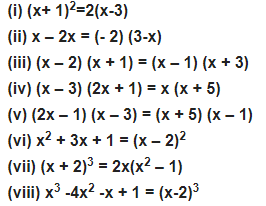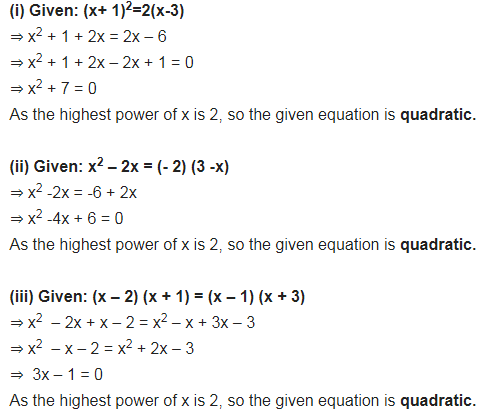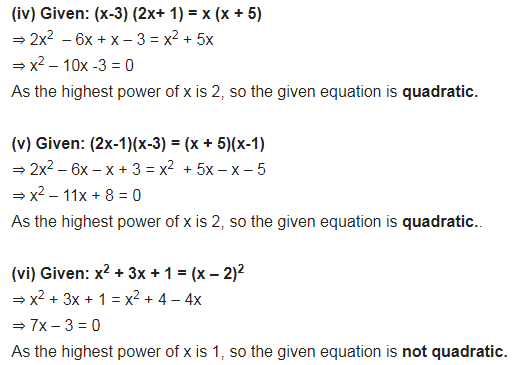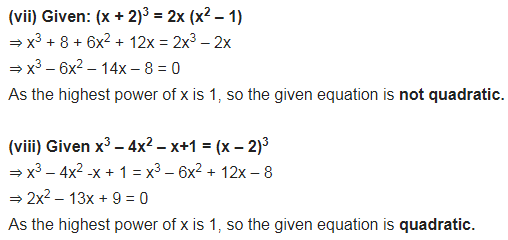# Check whether the following are quadratic equations:

Check whether the following are quadratic equations: